# How to Find a Fraction of a Number

How to Find a Fraction of a Number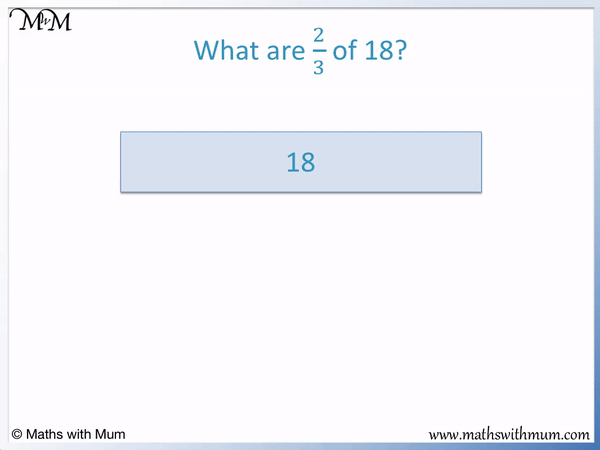• We will use this bar model to find   2/3   of 18.
• We want to find two thirds of 18.
• We can find one third of 18 by dividing by 3.
• 18 ÷ 3 = 6 and so, one third of 18 is 6.
• We want two thirds, so we multiply one third by 2.
• 6 × 2 = 12 and so,   2/3   of 18 = 12.
• We divided by the number on the bottom of the fraction and multiplied by the number on the top of the fraction.
Multiply by the numerator on top and divide by the denominator on the bottom.• We want to find   3/4   of 20.
• To find a fraction of an amount, divide the amount by the denominator on the bottom and then multiply by the numerator on top.
• Dividing by the denominator: 20 ÷ 4 = 5.
• Multiplying by the numerator: 5 × 3 = 15.
• 3/4   of 20 = 15.
• We can also multiply by the numerator first and then divide by the denominator afterwards to get the same answer.
• Multiplying by the numerator: 20 × 3 = 60.
• Dividing by the denominator: 60 ÷ 4 = 15.Supporting Lessons# Finding a Fraction of a Number

## How to Find a Fraction of a Number

To find a fraction of a number, follow these steps:

1. Divide the number by the denominator of the fraction.
2. Multiply this result by the numerator of the fraction.

For example, to calculate   3/4   of 20, divide 20 by 4 to get 5 and then multiply 5 by 3 to get 15.   3/4   of 20 = 15.

We can see these steps more clearly using the bar model shown below.

The first step is to divide the number by the denominator.

20 ÷ 4 = 5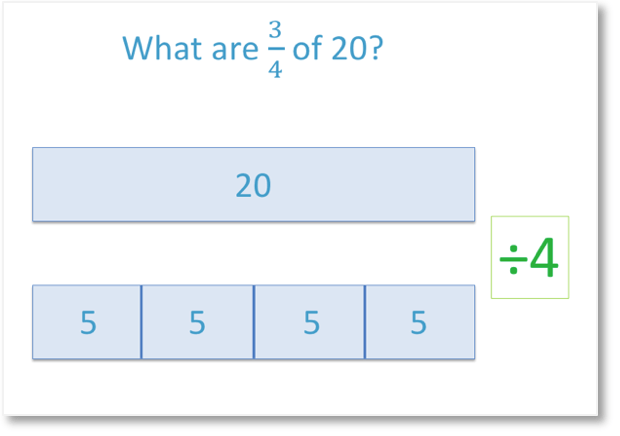One quarter of 20 is 5. We know this because five fours make twenty.

The second step is to multiply this result by the numerator.

5 × 3 = 15

One quarter is 5, so three quarters are 15.We can see three of the quarters are shown shaded on our bar model.It does not matter in which order the steps for finding a fraction of a number are done. We can multiply by the numerator first and then divide by the denominator to get the same result.

For example, in   3/4   of 20, we can multiply 20 by the numerator of 3 first.

3 × 20 = 60

Then we can divide this result by the denominator of 4.

60 ÷ 4 = 15

The same result is found whether we multiply or divide first.

When finding the fraction of an amount, it is best to divide by the denominator and then multiply by the numerator afterwards. This is because this order results in multiplying and dividing smaller numbers which is less likely to result in a mistake.

A unit fraction is a fraction with a numerator of 1. A non-unit fraction is any fraction where the numerator is greater than 1.

Here are some further examples of finding a non-unit fraction of a number.To find   23 , divide by 3 and then multiply by 2.

To find 34 , divide by 4 and then multiply by 3.

To find 45 , divide by 5 and then multiply by 4.23 of 18 = 12.

34 of 20 = 15.

45 of £30 = £24.

## How to Find a Fraction of an Amount

To find a fraction of an amount, simply divide the amount by the denominator on the bottom of the fraction and then multiply this result by the numerator on the top of the fraction.

If there are units in the total amount, simply ignore these for the calculation and then add them back in afterwards.

For example here is the question asking us to find   3/5   of 25 grams.We first divide 25 by 5 to get 5 and then we multiply this result by 3 to get 15.

3/5   of 25 g = 15 g.

Here is an example of finding a fraction of an amount of money . We have the question   4/5   of £30.We first divide the amount by the denominator on the bottom of the fraction.

30 ÷ 5 = 6

We then multiply this result by the numerator.

6 × 4 = 244/5   of £30 = £24.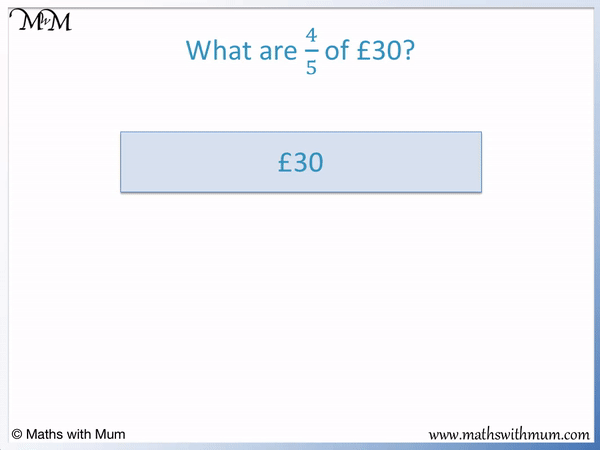Here is another example of finding a fraction of an amount.

In this question we are finding a fraction of a length. What is   2/7   of 56 cm?

First, divide the amount by the denominator. 56 ÷ 7 = 8.

Secondly, multiply this result by the numerator. 8 × 2 = 16.2/7   of 56 cm = 16 cm.

Here is an example of finding a fraction of a volume.

What is   5/6   of 42 ml?

Firstly, divide the amount by the denominator. 42 ÷ 6 = 7.

Secondly, multiply this result by the numerator. 7 × 5 = 35.5/6   of 42 ml = 35 ml.

## Fractions of Amounts using the Bar Model

A bar model is simply a rectangle drawn to represent the total amount. To find a fraction of an amount using the bar model, simply divide the total bar into smaller rectangles of equal size and then shade in however many of these parts you need.

The denominator of the fraction is how many parts the bar model is split into.

The numerator of the fraction is how many parts of the bar model to be shaded.

Here are the steps for finding a fraction of an amount using a bar model.

1. Draw a rectangular bar to represent the total amount.
2. Divide this bar into the same number of equally sized parts as the denominator of the fraction.
3. Write the value found by dividing the total by the denominator inside each part.
4. Shade in as many of these parts as the numerator of the fraction.
5. Multiply the number of parts shaded by the value of each part.

Here is an example of using the bar model to work out the fraction of an amount   2/3   of 18.

Firstly, a rectangular bar is drawn to represent the total. 18 is written inside the bar.The next step is to divide the bar into the same number of parts as the denominator of the fraction.

The denominator is 3 so the bar model is divided into 3 equal parts.

18 ÷ 3 = 6, so we write 6 inside each part.

The next step is to shade in as many parts as there are in the numerator of the fraction.

The numerator is 2, so we shade in 2 parts.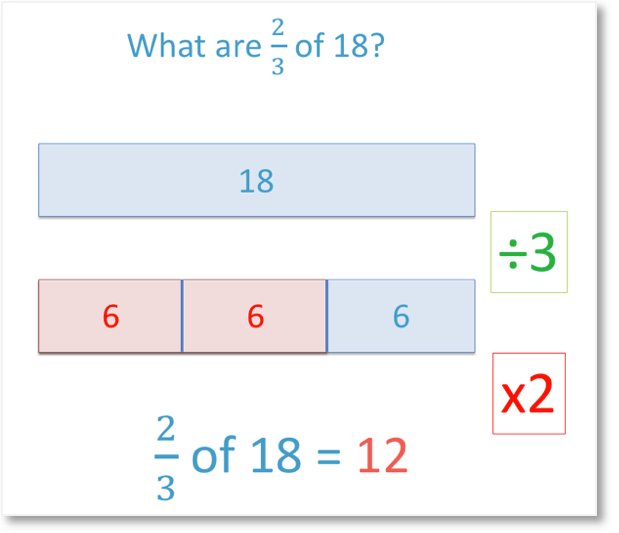The final step is to multiply the number of parts by the value of each part.

2 × 6 = 12 and so   2/3   of 18 = 12.The bar model method is used to introduce the written method for finding fractions of amounts. The bar model method allows the size of a fraction to be visualised, which can make the process of dividing by the denominator and multiplying by the numerator more understandable.

When teaching how to find fractions of amounts, it is best to start with finding unit fractions of an amount. This is because finding a unit fraction of an amount is a simple one-step division. Once this is understood, non-unit fractions of an amount can be found by multiplying the result by the numerator.

The bar model method is a visual way to introduce non-unit fractions as the numerator of the fraction is represented by the number of parts shown. It is best to show the bar model method before going straight into a written method, so that the conceptual understanding of what a fraction of an amount is is first understood.

## How to Find an Improper Fraction of a Number

To find an improper fraction of a number, use the same method used to find a proper fraction of a number. Divide the number by the denominator and then multiply this by the numerator. When finding an improper fraction of a number, the answer will be bigger than the original number.

An improper fraction is simply a fraction that has a larger numerator on top than its denominator on the bottom. It behaves in exactly the same way as a proper fraction does.

For example, we will work out   5/2   of 10.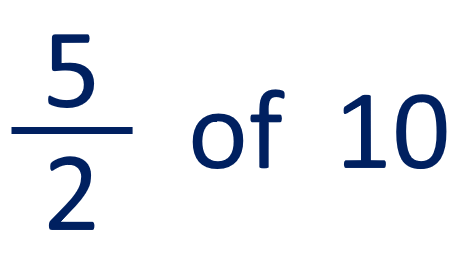The steps for finding an improper fraction of a number are:

1. Divide the number by the denominator.
2. Multiply this result by the numerator.

10 ÷ 2 = 5 and then 5 × 5 = 25.5/2   of 10 is 25. We can see that finding an improper fraction of a number makes the original number get larger.

This is the opposite of finding a proper fraction of a number, which makes the original number get smaller.

## How to Find a Mixed Number Fraction of a Number

To find a mixed number fraction of a number, first convert the mixed fraction into an improper fraction. Then divide the number by the denominator of the fraction and multiply this result by the numerator. Finding a mixed fraction of a number will make the original number larger.

For example, here is the question of 3   1/2   of 4.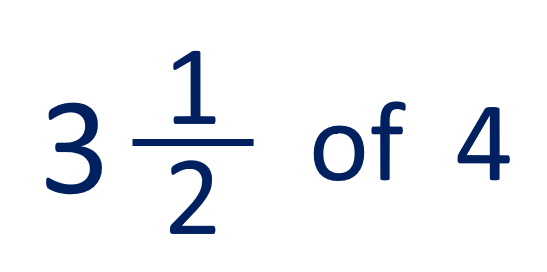A mixed number is a combination of a whole number and a fraction. 3   1/2   is a mixed number because it is made up of the whole number 3 and the fraction   1/2  .

The first step is to convert the mixed number into an improper fraction.

To convert a mixed number into an improper fraction:

• Multiply the denominator and the whole number together.
• Add the numerator of the fraction to this result to get the numerator of the improper fraction.
• Keep the denominator the same.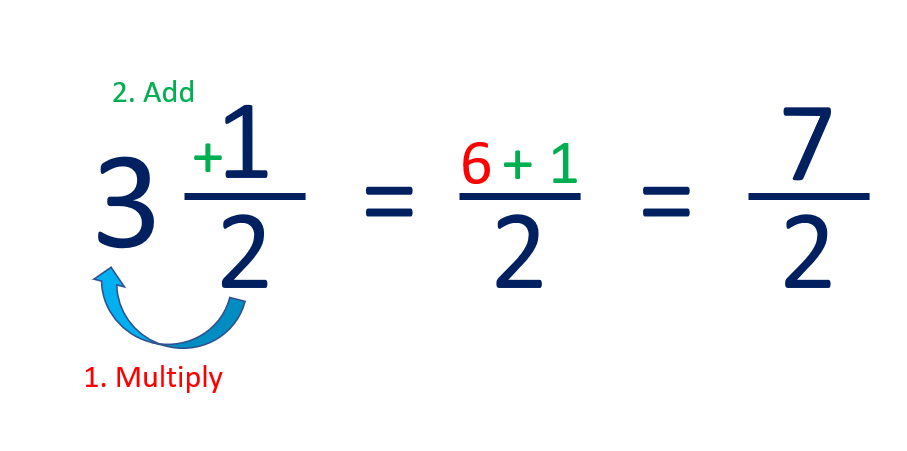We keep the denominator as 2.

We multiply the denominator of 2 by the whole number of 3 to get 6.

We add the numerator of 1 to this to get 7, which is the numerator of our answer.

3   1/2   =   7/2  .

Now that we have converted the mixed number to an improper fraction, we can find the fraction of the number.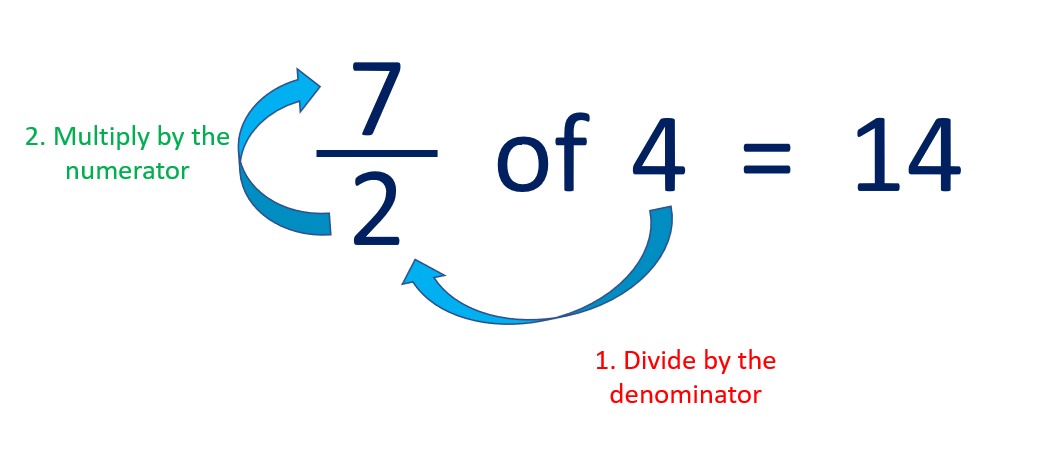We divide by the denominator and then multiply by the numerator.

4 ÷ 2 = 2 and then 2 × 7 = 14.

3   1/2   of 4 = 14.

Again we can see that finding a mixed number fraction of a number made it larger.

Alternatively, to multiply a mixed number by a whole number, simply multiply by the whole number part and the fraction part separately before adding the results together.

3   1/2   is made up of 3 and   1/2  .

We can multiply 4 by 3 and then by   1/2  .

4 × 3 = 12 and 4 ×   1/2   = 2.

12 + 2 = 14, which is the same answer as using the other method.

## How to Find a Fraction of a Decimal Number

To find a fraction of a decimal number, divide the decimal by the denominator and multiply this result by the numerator.

For example, here is the decimal number of 3.6. We will find   2/3   of 3.6.To find a fraction of a decimal:

• Divide the decimal by the denominator.
• Multiply this result by the numerator.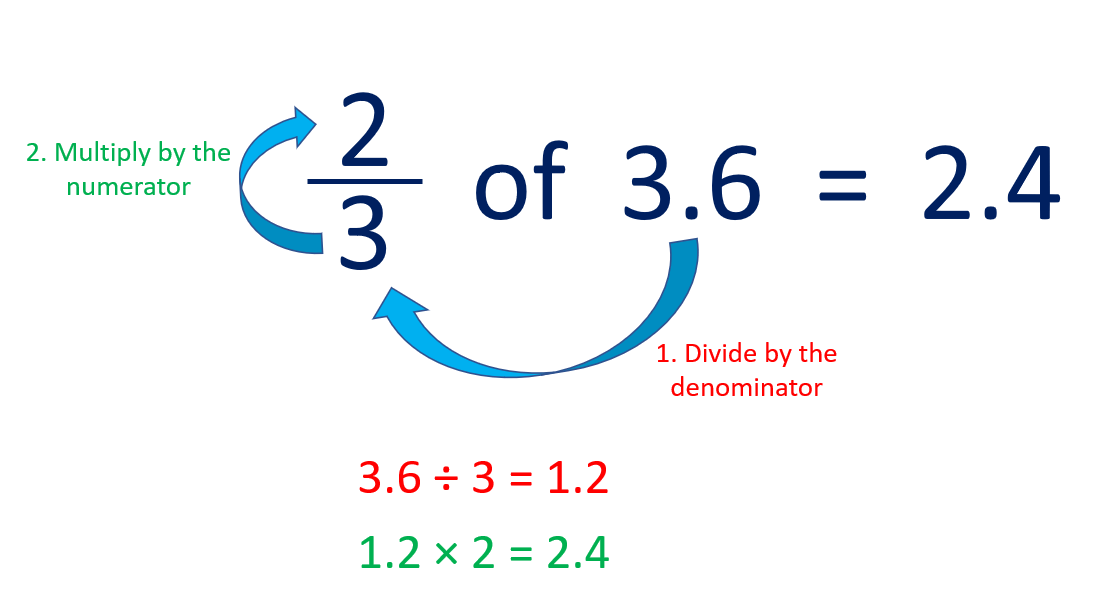3.6 ÷ 3 = 1.2 and then 1.2 × 2 = 2.4

2/3   of 3.6 = 2.4.

## How to Find a Fraction of a Fraction

To find a fraction of a fraction, multiply the numerators together to find the numerator of the answer and then multiply the denominators together to find the denominator of the answer. Finding a fraction of a fraction is the same as multiplying fractions.

In maths, the word 'of' can mean to multiply.

Finding a fraction of a fraction simply means to multiply the first fraction by the second fraction.

For example, here is   2/5   of   3/4  .

We replace the word 'of' with a multiplication sign. We write   2/5   of   3/4   as   2/5   ×   3/4  .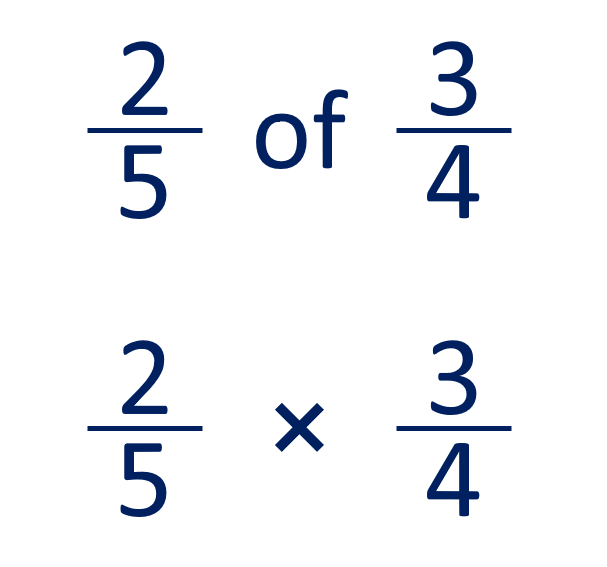To find a fraction of a fraction, the first step is to multiply the numerators together to find the numerator of the answer.

The next step is to multiply the denominators together to find the denominator of the answer.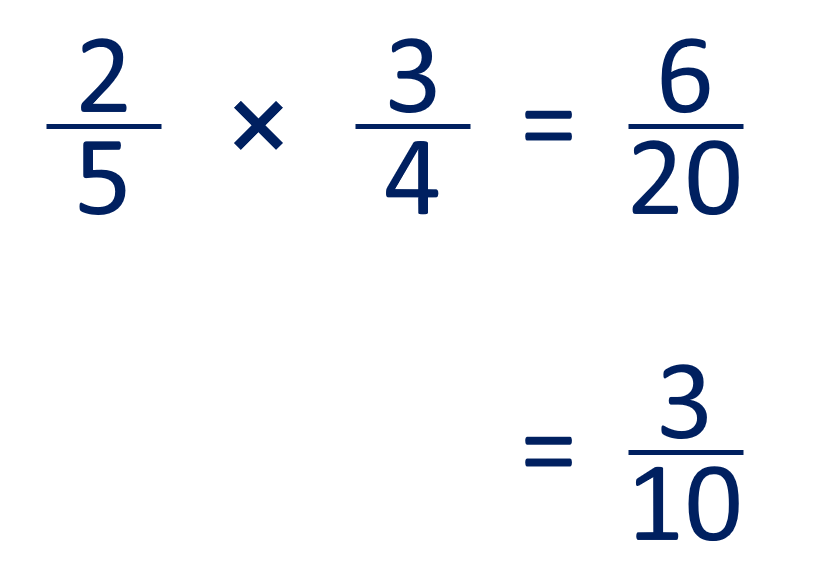Multiplying the numerators: 2 × 3 = 6.

Multiplying the denominators: 5 × 4 = 20.

2/5   ×   3/4   =   6/20  .

We then simplify the fraction if possible. Both 6 and 20 are even and can be divided by 2.

6/20   =   3/10  .

Therefore   2/5   of   3/4   =   3/10  .Now try our lesson on Adding Fractions with Common Denominators where we learn how to add two fractions that have the same denominator as each other.